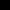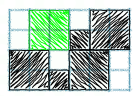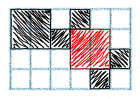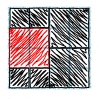### Problem Statement

Two people are playing a game, taking turns filling in squares on a piece of graph paper. On each turn, they can either fill in a single empty square or fill in a 2x2 block of 4 empty squares. Once all the squares in the grid are filled, the last player to have filled in a square is the winner.

The only restriction is that if we number the rows from top to bottom starting at zero, the top half of every 2x2 block that a player fills in during a turn must lie in an even-numbered row. For example, the following moves (in green) are legal:while the following moves (in red) are illegal:The current state of the grid will be given as a String[] state. Each element of state will be one row of the grid, and each character of each element of state will represent one square in the row. A '.' (period) represents an empty square, and a '#' represents a filled-in square.

From this current state, determine who can win the game assuming both people play optimally. If the next player to move can win return the String "first", otherwise, return the String "second" (all quotes for clarity).

### Definition

 Class: LittleSquares Method: winner Parameters: String[] Returns: String Method signature: String winner(String[] state) (be sure your method is public)

### Constraints

-states will contain an even number of elements, between 2 and 10, inclusive.
-The length of each element of states will be even and between 2 and 10, inclusive.
-The length of each element of states will be the same.
-Each character in states will be '.' (period) or '#'.

### Examples

0)

 ```{ "..", ".." }```
`Returns: "first"`
 On an empty 2x2 grid, the first player can win the game with a single move.
1)

 ```{ "...#", "..##" }```
`Returns: "first"`
 If the first player draws a 2x2 square on the left side of the grid, she will lose. Likewise if she draws a 1x1 square in the rightmost open space. However if she draws a 1x1 square in any of the other four empty spaces, that will leave 4 empty squares to be filled in and she will be able to move last.
2)

 ```{ "..", "..", "..", ".." }```
`Returns: "second"`
 If the first player draws a 2x2 square on her first move, the second player will draw another 2x2 square and win. If the first player draws a 1x1 square first, the second player will draw another in the other half of the grid, leaving 6 empty spaces that can only be filled in one at a time.
3)

 ```{ "....", "...." }```
`Returns: "first"`
 The first player can draw a 2x2 square in the middle of the grid, leaving 4 empty spaces.
4)

 ```{ ".##.", "#..#", "#..#", ".##." }```
`Returns: "second"`
5)

 ```{ "#.......", ".....##.", ".....##.", "........", "........", "........", "........", "#......#" }```
`Returns: "first"`

#### Problem url:

http://www.topcoder.com/stat?c=problem_statement&pm=8543

#### Problem stats url:

http://www.topcoder.com/tc?module=ProblemDetail&rd=11123&pm=8543

legakis

#### Testers:

PabloGilberto , Olexiy , Andrew_Lazarev , ivan_metelsky

#### Problem categories:

Dynamic Programming